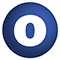# math

### Related Doc: package util

#### object math

Source
math.scala
Linear Supertypes
Ordering
1. Alphabetic
2. By Inheritance
Inherited
1. math
2. AnyRef
3. Any
1. Hide All
2. Show All
Visibility
1. Public
2. All

### Value Members

1. #### final def !=(arg0: Any): Boolean

Definition Classes
AnyRef → Any
2. #### final def ##(): Int

Definition Classes
AnyRef → Any
3. #### final def ==(arg0: Any): Boolean

Definition Classes
AnyRef → Any
4. #### final def asInstanceOf[T0]: T0

Definition Classes
Any
5. #### def clone(): AnyRef

Attributes
protected[java.lang]
Definition Classes
AnyRef
Annotations
@throws( ... )
6. #### final def eq(arg0: AnyRef): Boolean

Definition Classes
AnyRef
7. #### def equals(arg0: Any): Boolean

Definition Classes
AnyRef → Any
8. #### def finalize(): Unit

Attributes
protected[java.lang]
Definition Classes
AnyRef
Annotations
@throws( classOf[java.lang.Throwable] )
9. #### final def getClass(): Class[_]

Definition Classes
AnyRef → Any
10. #### def hashCode(): Int

Definition Classes
AnyRef → Any
11. #### final def isInstanceOf[T0]: Boolean

Definition Classes
Any
12. #### final val lnOf2: Double

Natural log of 2

13. #### def log2(x: Double): Double

Calculates the base 2 logarithm of the given argument.

Calculates the base 2 logarithm of the given argument.

returns

a number such that 2nr is equal to our argument.

14. #### final def ne(arg0: AnyRef): Boolean

Definition Classes
AnyRef
15. #### def nextPowerOf2(nr: Long): Long

Given a positive long, returns the next power of 2 that is bigger than our argument, or the maximum that this function can return which is 262 (or 4,611,686,018,427,387,904).

Given a positive long, returns the next power of 2 that is bigger than our argument, or the maximum that this function can return which is 262 (or 4,611,686,018,427,387,904).

returns

a long that is a power of 2 and that is "closest" to the given argument.

16. #### def nextPowerOf2(nr: Int): Int

Given a positive integer, returns the next power of 2 that is bigger than our argument, or the maximum that this function can return which is 230 (or 1,073,741,824).

Given a positive integer, returns the next power of 2 that is bigger than our argument, or the maximum that this function can return which is 230 (or 1,073,741,824).

returns

an integer that is a power of 2, that is bigger than our argument and that is "closest" to it.

17. #### final def notify(): Unit

Definition Classes
AnyRef
18. #### final def notifyAll(): Unit

Definition Classes
AnyRef
19. #### def roundToPowerOf2(nr: Long): Long

Given a long, rounds it to the nearest power of two.

Given a long, rounds it to the nearest power of two. Note that the maximum that this function can return is 262 (or 4,611,686,018,427,387,904).

returns

a long that is a power of 2 and that is "closest" to the given argument.

20. #### def roundToPowerOf2(nr: Int): Int

Given a positive integer, rounds it to the nearest power of two.

Given a positive integer, rounds it to the nearest power of two. Note that the maximum that this function can return is 230 (or 1,073,741,824).

returns

an integer that is a power of 2 and that is "closest" to the given argument.

21. #### final def synchronized[T0](arg0: ⇒ T0): T0

Definition Classes
AnyRef
22. #### def toString(): String

Definition Classes
AnyRef → Any
23. #### final def wait(): Unit

Definition Classes
AnyRef
Annotations
@throws( ... )
24. #### final def wait(arg0: Long, arg1: Int): Unit

Definition Classes
AnyRef
Annotations
@throws( ... )
25. #### final def wait(arg0: Long): Unit

Definition Classes
AnyRef
Annotations
@throws( ... )